## Tamilnadu Samacheer Kalvi 10th Maths Solutions Chapter 3 Algebra Ex 3.15

10th Maths Exercise 3.15 Solutions Question 1.
Graph the following quadratic equations and state their nature of solutions,
(i) x2 – 9x + 20 = 0
Solution: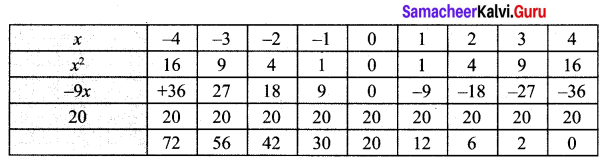Step 1:
Points to be plotted : (-4, 72), (-3, 56), (-2, 42), (-1, 30), (0, 20), (1, 12), (2, 6), (3, 2), (4, 0)
Step 2:
The point of intersection of the curve with x axis is (4, 0)
Step 3: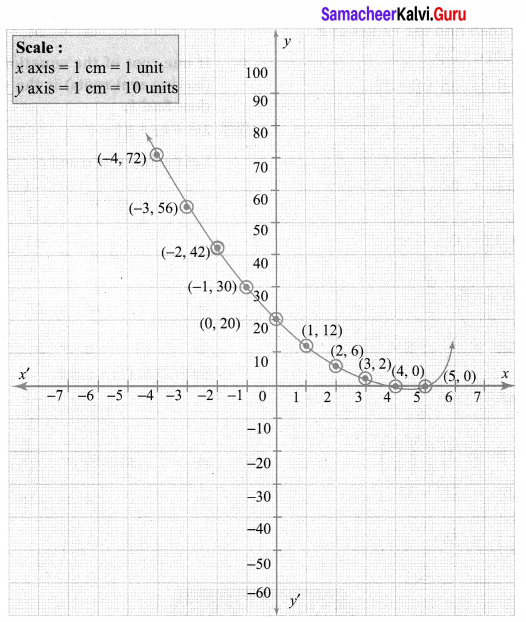The roots are real & unequal
∴ Solution {4, 5}

(ii) x2 – 4x + 4 = 0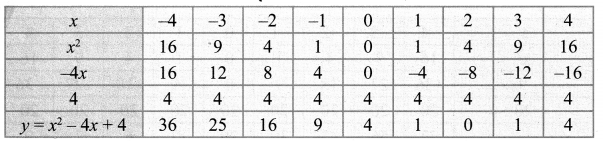Step 1: Points to be plotted : (-4, 36), (-3, 25), (-2, 16), (-1, 9), (0, 4), (1, 1), (2, 0), (3, 1), (4, 4)
Step 2: The point of intersection of the curve with x axis is (2, 0)
Step 3: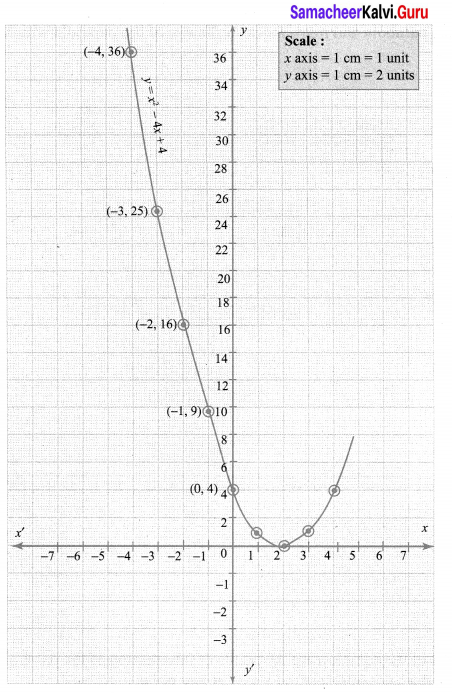Since there is only one point of intersection with x axis, the quadratic equation x2 – 4x + 4 = 0 has real and equal roots.
∴ Solution{2, 2}

(iii) x2 + x + 7 = 0
Let y = x2 + x + 7
Step 1: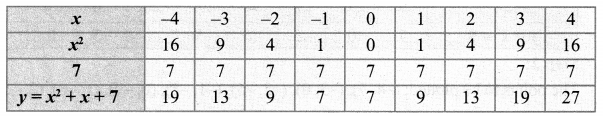Step 2:
Points to be plotted: (-4, 19), (-3, 13), (-2, 9), (-1, 7), (0, 7), (1, 9), (2, 13), (3, 19), (4, 27)
Step 3:
Draw the parabola and mark the co-ordinates of the parabola which intersect with the x-axis.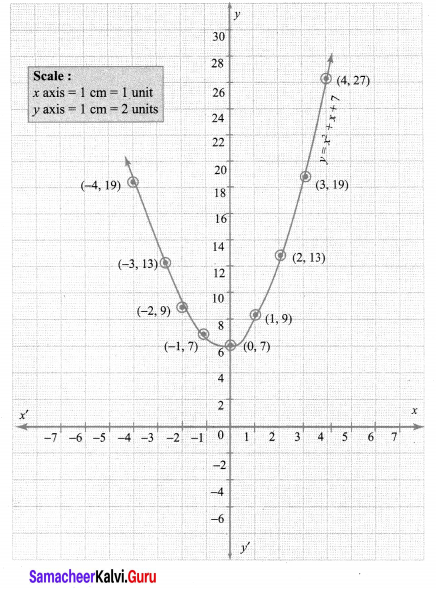Step 4:
The roots of the equation are the points of intersection of the parabola with the x axis. Here the parabola does not intersect the x axis at any point.
So, we conclude that there is no real roots for the given quadratic equation,

(iv) x2 – 9 = 0
Let y = x2 – 9
Step 1: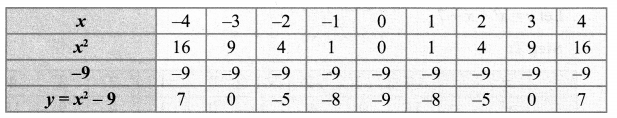Step 2:
The points to be plotted: (-4, 7), (-3, 0), (-2, -5), (-1, -8), (0, -9), (1,-8), (2, -5), (3, 0), (4, 7)
Step 3:
Draw the parabola and mark the co-ordinates of the parabola which intersect the x-axis.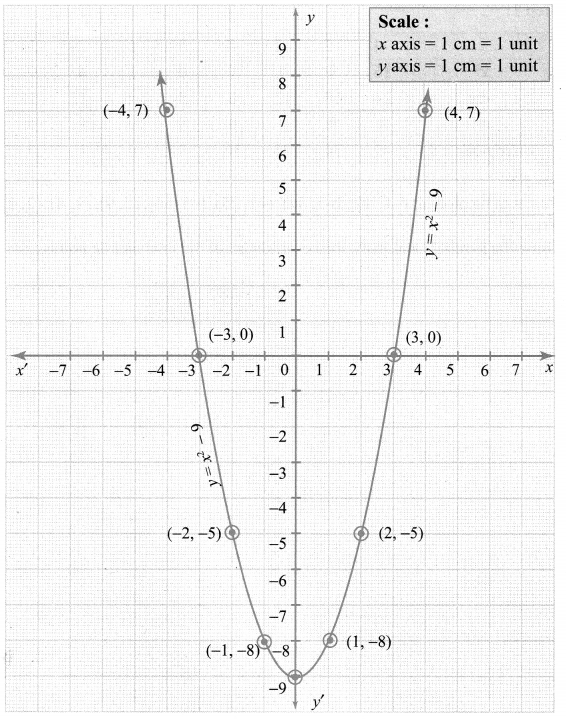Step 4:
The roots of the equation are the co-ordinates of the intersecting points (-3, 0) and (3, 0) of the parabola with the x-axis which are -3 and 3 respectively.
Step 5:
Since there are two points of intersection with the x axis, the quadratic equation has real and unequal roots.
∴ Solution{-3, 3}

(v) x2 – 6x + 9 = 0
Let y = x2 – 6x + 9
Step 1: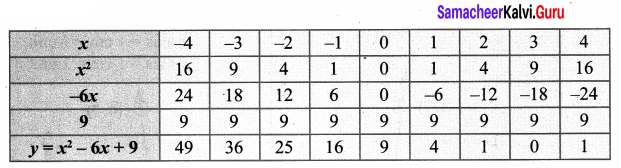Step 2:
Points to be plotted: (-4, 49), (-3, 36), (-2, 25), (-1, 16), (0, 9), (1, 4), (2, 1), (3, 0), (4, 1)
Step 3:
Draw the parabola and mark the co-ordinates of the intersecting points.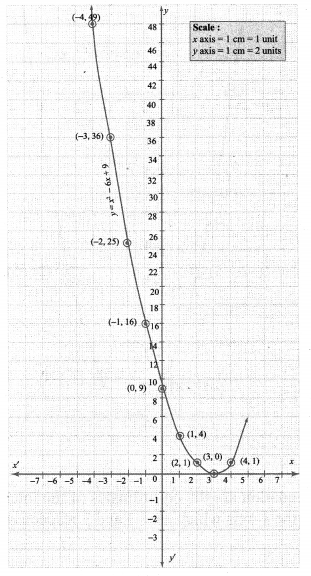Step 4:
The point of intersection of the parabola with x axis is (3, 0)
Since there is only one point of intersection with the x-axis, the quadratic equation has real and equal roots. .
∴ Solution (3, 3)

(vi) (2x – 3)(x + 2) = 0
2x2 – 3x + 4x – 6 = 0
2x2 + 1x – 6 = 0
Let y = 2x2 + x – 6 = 0
Step 1: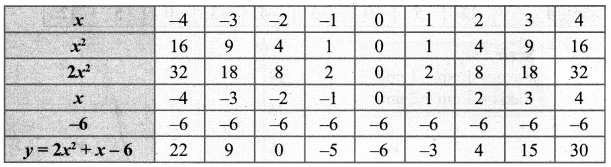Step 2:
The points to be plotted: (-4, 22), (-3, 9), (-2, 0), (-1, -5), (0, -6), (1, -3), (2, 4), (3, 15), (4, 30)
Step 3:
Draw the parabola and mark the co-ordinates of the intersecting point of the parabola with the x-axis.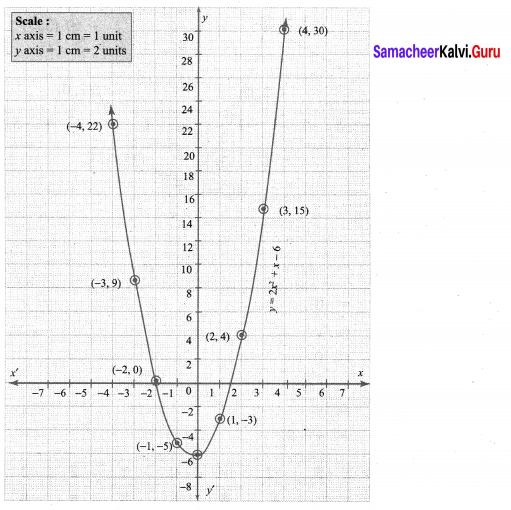Step 4:
The points of intersection of the parabola with the x-axis are (-2, 0) and (1.5, 0).
Since the parabola intersects the x-axis at two points, the, equation has real and unequal roots.
∴ Solution {-2, 1.5}

Question 2.
Draw the graph of y = x2 – 4 and hence solve x2 – x – 12 = 0
Solution: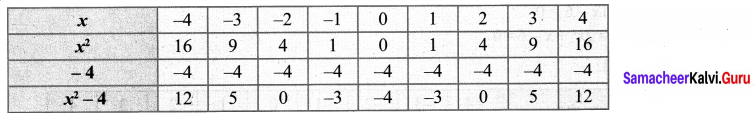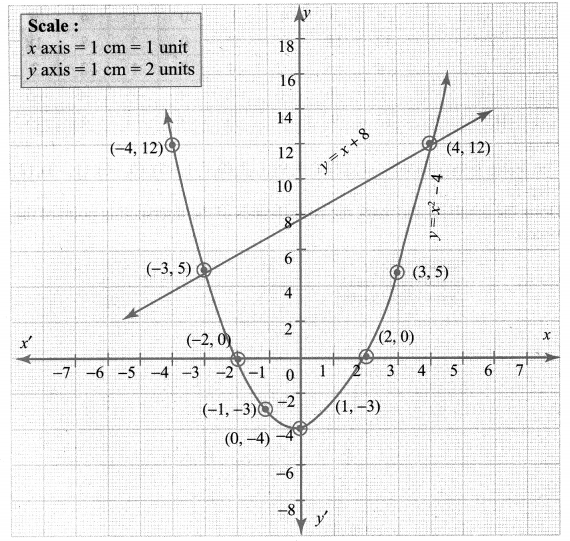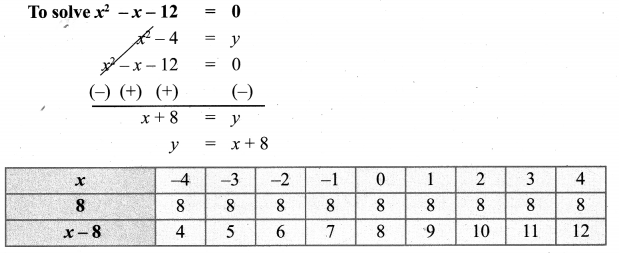Point of intersection (-3, 5), (4, 12) solution of x2 – x – 12 = 0 is -3, 4

10th Maths Graph 3.15 Answers Question 3.
Draw the graph of y = x2 + x and hence solve x2 + 1 = 0.
Solution: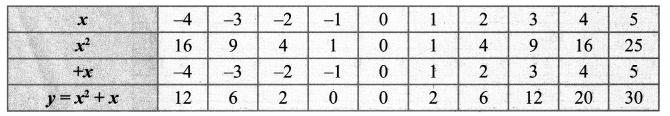Draw the parabola by the plotting the points (-4, 12), (-3, 6), (-2, 2), (-1, 0), (0, 0), (1, 2), (2, 6), (3, 12), (4, 20), (5, 30)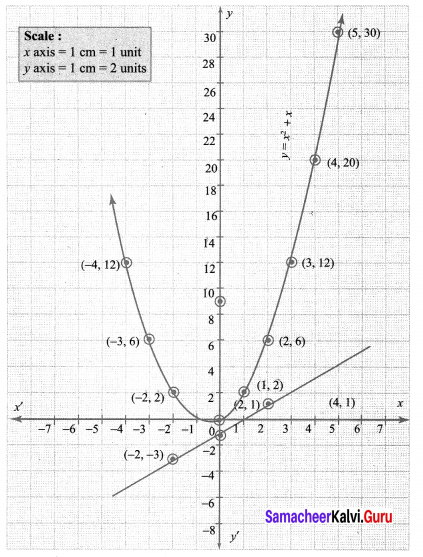To solve: x2 + 1 = 0, subtract x2 + 1 = 0 from y = x2 + x.
x2 + 1 = 0 from y = x2 + x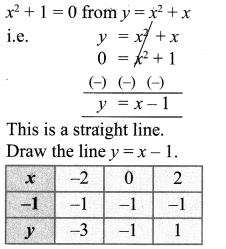Plotting the points (-2, -3), (0, -1), (2, 1) we get a straight line. This line does not intersect the parabola. Therefore there is no real roots for the equation x2 + 1 = 0.

10th Maths Guide Graph Question 4.
Draw the graph of y = x2 + 3x + 2 and use it to solve x2 + 2x + 1 = 0.
Solution: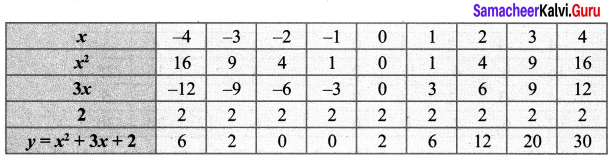Draw the parabola by plotting the point (-4, 6), (-3, 2), (-2, 0), (-1, 0), (0, 2), (1, 6), (2, 12), (3, 20), (4, 30).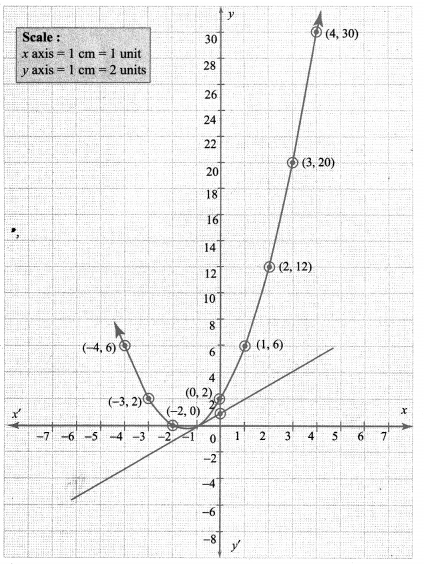To solve x2 + 2x + 1 = 0, subtract x2 + 2x + 1 = 0 from y = x2 + 3x + 2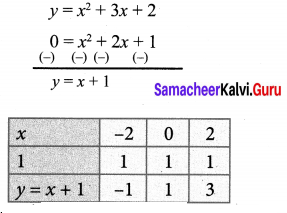Draw the straight line by plotting the points (-2, -1), (0, 1), (2, 3)
The straight line touches the parabola at the point (-1,0)
Therefore the x coordinate -1 is the only solution of the given equation

10th Graph Exercise 3.15 Solutions Question 5.
Draw the graph of y = x2 + 3x – 4 and hence use it to solve x2 + 3x – 4 = 0. y = x2 + 3x – 4
Solution: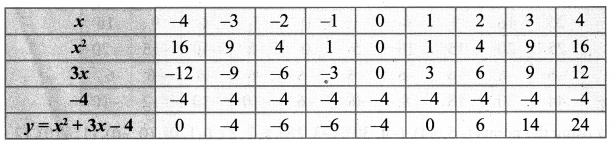Draw the parabola using the points (-4, 0), (-3, -4), (-2, -6), (-1, -6), (0, -4), (1, 0), (2, 6), (3, 14), (4, 24).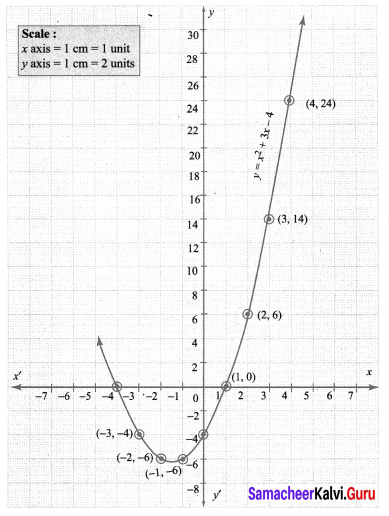To solve: x2 + 3x – 4 = 0 subtract x2 + 3x – 4 = 0 from y = x2 + 3x – 4 ,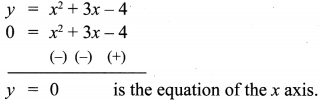The points of intersection of the parabola with the x axis are the points (-4, 0) and (1, 0), whose x – co-ordinates (-4, 1) is the solution, set for the equation x2 + 3x – 4 = 0.

10th Maths Graph Answers Question 6.
Draw the graph of y = x2 – 5x – 6 and hence solve x2 – 5x – 14 = 0.
Solution: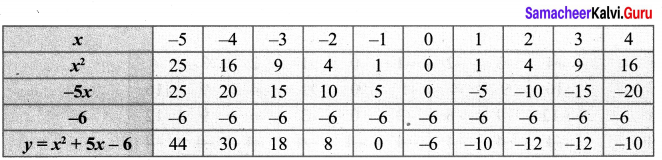Draw the parabola using the points (-5, 44), (-4, 30), (-3, 18), (-2, 8), (-1, 0), (0, -6), (1, -10), (2, -12), (3, -12), (4, -10)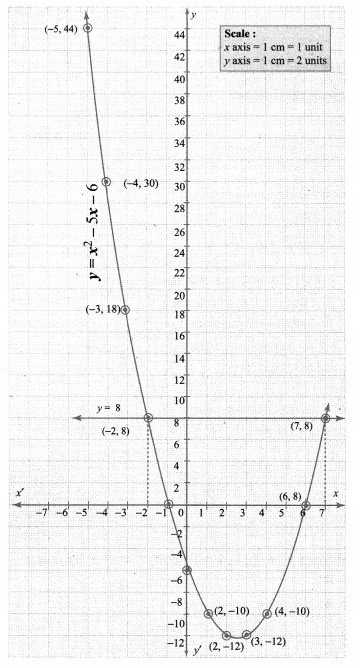To solve the equation x2 – 5x – 14 = 0, subtract x2 – 5x – 14 = 0 from y = x2 – 5x – 6.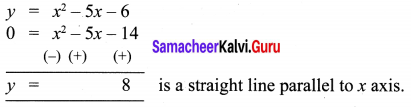The co-ordinates of the points of intersection of the line and the parabola forms the solution set for the equation x2 – 5x – 14 = 0.
∴ Solution {-2, 7}

10th Class Maths Graph Pdf Question 7.
Draw the graph of y = 2x2 – 3x – 5 and hence solve 2x2 – 4x – 6 = 0. y = 2x2 – 3x – 5
Solution: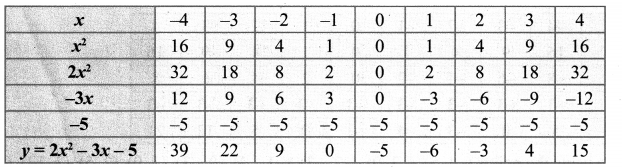Draw the parabola using the points (-4, 39), (-3, 22), (-2, 9), (-1, 0), (0, -5), (1, -6), (2, -3), (3, 4), (4, 15).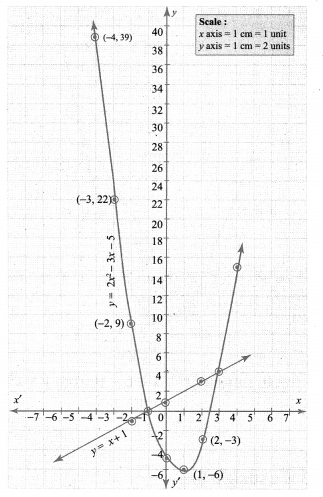To solve 2x2 – 4x – 6 = 0, subtract it from y = 2x2 – 3x – 5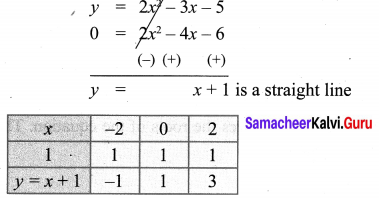Draw a straight line using the points (-2, -1), (0, 1), (2, 3). The points of intersection of the parabola and the straight line forms the roots of the equation.
The x-coordinates of the points of intersection forms the solution set.
∴ Solution {-1, 3}

10th Maths Graph Question 8.
Draw the graph of y = (x – 1)(x + 3) and hence solve x2 – x – 6 = 0.
Solution:
y = (x – 1)(x + 3) = x2 – x + 3x – 3 = 0
y = x2 + 2x – 3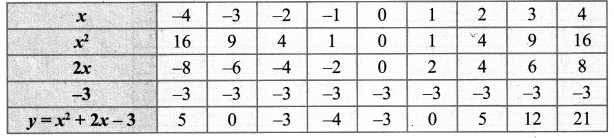Draw the parabola using the points (-4, 5), (-3, 0), (-2, -3), (-1,-4), (0, -3), (1, 0), (2, 5), (3, 12), (4, 21)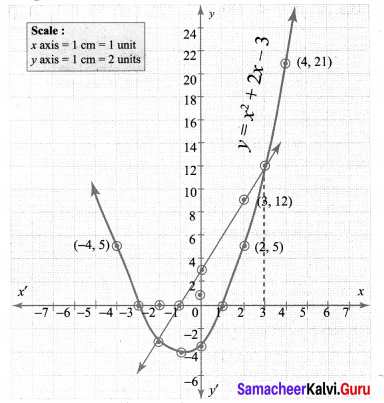To solve the equation x2 – x – 6 = 0, subtract x2 – x – 6 = 0 from y = x2 – 2x – 3.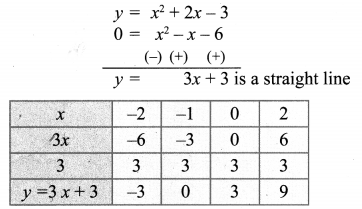Plotting the points (-2, -3), (-1, 0), (0, 3), (2, 9), we get a straight line.
The points of intersection of the parabola with the straight line gives the roots of the equation. The co¬ordinates of the points of intersection forms the solution set.
∴ Solution {-2, 3}